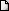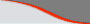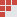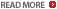# Algebra, Quadratic Equation : Free CAT practice questions : Ascent Education - CAT preparation coursesLATEST NEWS • Ascent Success Stories • TANCET 16 Classes @ Chennai • XAT 16 Classes @ ChennaiHome | TANCET Coaching Class | TANCET study material | Online TANCET Course | Contact us
 Ascent's Courses TANCET Coaching Chennai Online TANCET Course TANCET MBA Study Material CAT Classroom Chennai CAT Correspondence India Mock CAT Series GRE Coaching Chennai GMAT Coaching Chennai Online GMAT CourseUS B Schools Faculty List Jobs @ Ascent Testimonials Ascent Success Stories Contact Us +91 44 4500 8484 +91 96000 48484
You are here: Home » CAT Math » Algebra » Quadratic equations - roots

#### Quadratic Equation : CAT 2016 Preparation Questions

Question 4 the day : April 15, 2003
The question for the day is from the topic Algebra. It is about finding a root of a quadratic, given the equation and another root.

Question
If one of the roots of the quadratic equation 2x2 - 7x + q = 0 is 3, find the other root

(1) -3
(2) -1/2
(3) 1/2
(4) 1/4

Correct Choice is (3) and Correct Answer is 1/2

Substituting x = 3 in the given equation we get
2(32) - 7 (3) + q = 0. Therefore, q = 3

Now, the given equation becomes 2x2 - 7x + 3 = 0

By solving this we get (x - 3) (2x - 1) = 0
i.e., x = 3 & 1/2. The second root is 1/2.

## More Questions on Linear, Quadratic and Cubic Equations - Algebra

 5. 6. 7.

## XLRI XAT TANCET Practice Question Samples

 TANCET MBA MCA Practice Questions with detailed explanation and shortcuts.Comprehensive study material for GMAT Quant. 1800+ questions.